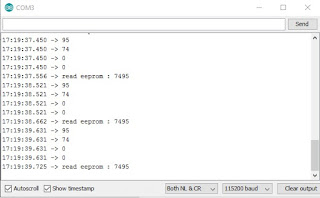## EEPROM

This is a bit different from standard EEPROM class. You need to call `EEPROM.begin(size)` before you start reading or writing, size being the number of bytes you want to use. Size can be anywhere between 4 and 4096 bytes.`EEPROM.write` does not write to flash immediately, instead you must call `EEPROM.commit()` whenever you wish to save changes to flash. `EEPROM.end()` will also commit, and will release the RAM copy of EEPROM contents.
EEPROM library uses one sector of flash located just after the SPIFFS.
Three examples included.
many problem when you want to write or read EEPROM for interger etc.
to break down data and store it on eeprom we have to consider the data and the amount of data that will be stored at an address
for example rtc data or counting that will be stored at eeprom 0 address. there is a maximum amount of data that must be considered. the solution is to break down the data
here example separate data for EEPROM
#include <EEPROM.h>
``````
int data1 = 7495 ; // data
int num1,num2,num3,num4;
void setup() {
EEPROM.begin(512);  // setup
Serial.begin(115200);
}

void loop() {

value = (value*1)+(value1*100)+(value2*10000)+(value3*1000000);
Serial.println(value); // show result read on eeprom
delay(1000);
num1 = (data1%100); // separate value or time
num2 = (data1/100)%100;
num3 = (data1/10000)%100;
num4 = (data1/1000000)%100;
Serial.println(num1);
Serial.println(num2);
Serial.println(num3);
Serial.println(num4);
//  int val  = 00;
//  int val1 = 00;
//  int val2 = 8;
//  int val3 = 1;
EEPROM.write(addr, num1); // save on eeprom

EEPROM.commit();

delay(100);
}``````
``````
``````Result
``````
I hope this can be useful and help you ... don't forget to share ... see you``````
``````Baca juga :Audio Spectrum Analyzer Arduino TUTORIAL CONTROL VTScada Lite ARDUINOTracking Kendaraan Arduino GPSMATLAB IMAGE PROCESSING DETEKSI WAJAH DENGAN METODE THRESHOLDING
``````